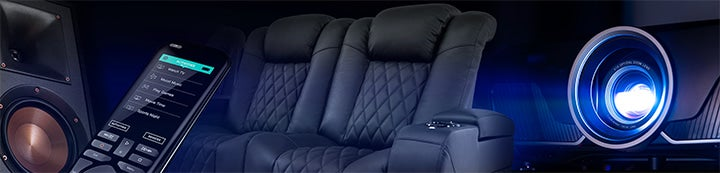1 - 3 of 3 Posts

#### Gibby Travis

·
##### Registered
Joined
·
29 Posts
Discussion Starter
I'm building a subwoofer for my friends art film theater. I'm trying to figure out how much amplifier power I need to drive the drivers to Xmax, but my calculations do not match those made by Win ISD. If Win ISD is right I need about 1000 W and can expect about 121 dB at one meter. If my calculations are right then I can expect about 116 dB at one meter and I need about 500 W to achieve it.

I'm using two Titanic Mk III drivers in a 15 ft³ box tuned to 19 Hz.

Driver Parameters:

fs= 24

Vas= 5.46 ft³ * 2 = 10.92 ft³ (5.46 ft³ per driver)

Qms= 6.75

Qes= 0.52

Qts= 0.49

Xmax= 20.5 mm

Vd≈ 77 in³ * 2 = 154 in³ = 0.00252 m³ (I estimated that the Vd was about 77 in³ per driver since Vd isn't given)

f3≈ 19 Hz

Efficiency:

ƞₒ= ( (2.7 * 10^-8) ( fs^3 * Vas) ) / Qes = 0.00784 W

where Vas is in ft³

112 + 10 * log 0.00784 = 91 dB SPL 1 W/1m

Max SPL:

Par= 3 * f3^4 * Vd^2 = 2.48 W

where Vd is in m³

112 + 10 * log 2.48 = 116 dB SPL @ 1m

Max Input Power:

Per= Par / ƞₒ = 317 W

where Par and ƞₒ are in Watts

Win ISD calculated efficiency at 91 dB 1W/1m which is exactly what I calculated.

Win ISD calculated Max SPL to be about 120 dB which is about 4 dB more than I did.

WIn ISD calculated Max Input Power to be about 875 W which is about 560 W more than what I figured.

What is going on here? Those seem like big discrepancies.

#### mtg90

·
##### Registered
Joined
·
2,185 Posts
•Gibby Travis

#### Gibby Travis

·
##### Registered
Joined
·
29 Posts
Discussion Starter
Thank you mtg90,

You are right I was off on the Vd. According to the spec sheet: http://www.parts-express.com/pdf/295-420s.pdf

Sd is 804.2 cm² which makes Vd about 0.0016 m³ * 2 or 0.0032 m³.

My calculations are still off from what Win ISD predicts though

I calculate max output to be about 118 dB SPL at one meter. Win ISD says about 122 dB. One is off by about 4dB that's a factor of about 2.5.

I calculate max input power to be about 510 Watts. Win ISD is now saying about 1470 Watts. Here somethings off by a factor of nearly 3.

The equations I'm using came from The Loudspeaker Design Cookbook (6th edition)

1 - 3 of 3 Posts